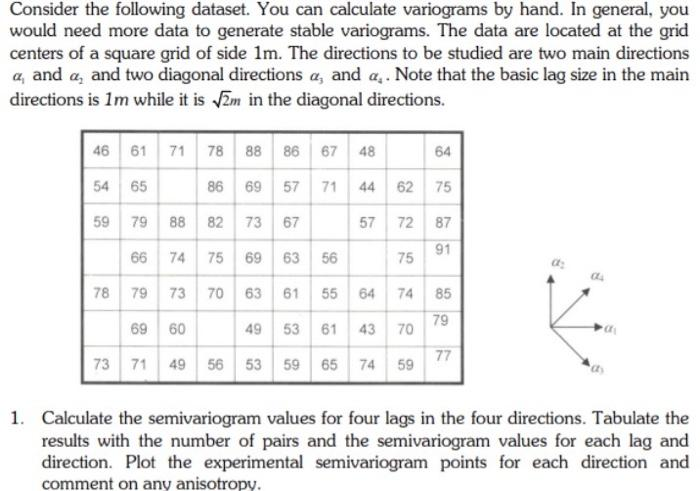Home / Expert Answers / Statistics and Probability / take-your-time-i-will-give-like-consider-the-following-dataset-you-can-calculate-variograms-by-hand-pa386

# (Solved): Take your time I will give like Consider the following dataset. You can calculate variograms by hand ...

Take your time I will give likeConsider the following dataset. You can calculate variograms by hand. In general, you would need more data to generate stable variograms. The data are located at the grid centers of a square grid of side . The directions to be studied are two main directions and and two diagonal directions and . Note that the basic lag size in the main directions is while it is in the diagonal directions. 1. Calculate the semivariogram values for four lags in the four directions. Tabulate the results with the number of pairs and the semivariogram values for each lag and direction. Plot the experimental semivariogram points for each direction and comment on any anisotropy.

We have an Answer from Expert